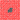## 互质点织成的布

Mon, 27th January 2020Edit on Github互质

# 问题起源

2009年11月， KeyTo9_Fans提问: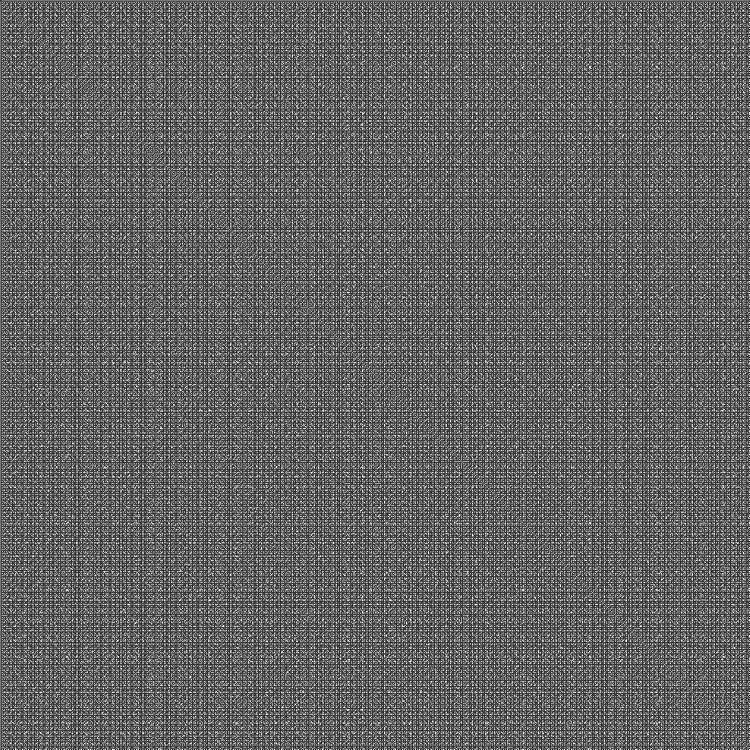## 问题一（经典问题）：

$[1,n]\times [1,n](n\to\infty)$的范围内，黑色方块所占的比例是否有极限？如何求这个极限值？

# 问题一证明

northwolves回复认为第一个问题的答案是$\frac6{\pi^2}$, 并且说这个问题他以前在CSDN上讨论过.
KeyTo9_Fans提供了他的思路:
P(N)有一个准确的上界：

$M=\sqrt{N}$，取下整。
$T=p_1p_2\dots p_n$

$\frac{p1^2-1}{p1^2}\frac{p2^2-1}{p2^2}\dots\frac{p_n^2-1}{p_n^2}$

（实际上可能不止M个完整的周期，但讨论的是上界，就放缩到$\frac M{M+1}$好了）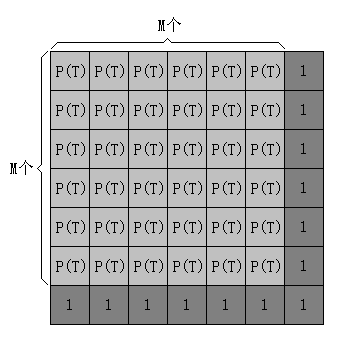p(n+1)、p(n+2)、......

$S = \frac1{{p^{\prime}}_1^2} + \frac1{{p^{\prime}}_2^2} + \frac1{{p^{\prime}}_3^2} + \dots$
$P(N)\gt P(T) - \frac3 M - S$

R·查特在1904年发现，两个随意写出的数中，互素的概率为$\frac6{\pi^2}$

mathe评论说如果一定要给这个证明鸡蛋挑骨头，那么就是没有证明概率的存在性。

# 问题二讨论

mathe说问题二中纯白色正方形区域可以任意大

$x\equiv -(i-1) (mod u_i) ,1\le i\le n$有解.

$y\equiv -(i-1) (mod v_i), 1\le i\le n$有解. 方阵$[x,x+n-1]\times[y,y+n-1]$满足条件.

# 问题三反例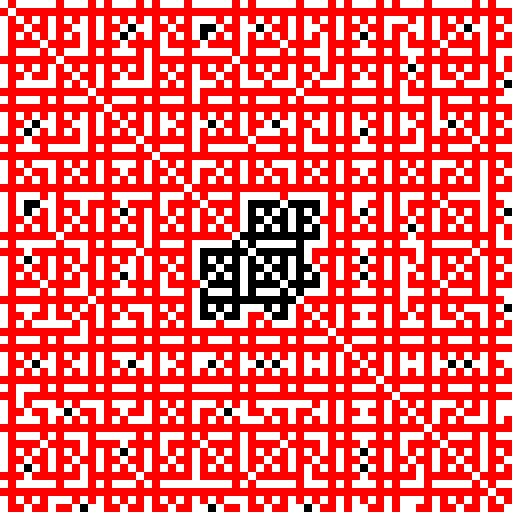# 问题二深入讨论计算

(x,y)，(x+1,y)，...，(x+n-1,y)
(x,y+1)，(x+1,y+1)，...，(x+n-1,y+1)
............
(x,y+n-1)，(x+1,y+n-1)，...，(x+n-1,y+n-1)

northwolves快速给出n=2： (14,20)
n=3: (1274 ,1308)
mathe建议对于更大的n只搜索特殊模式的解:

6 ? 2 3
? ? ? ?
2 ? 2 ?
3 ? ? 3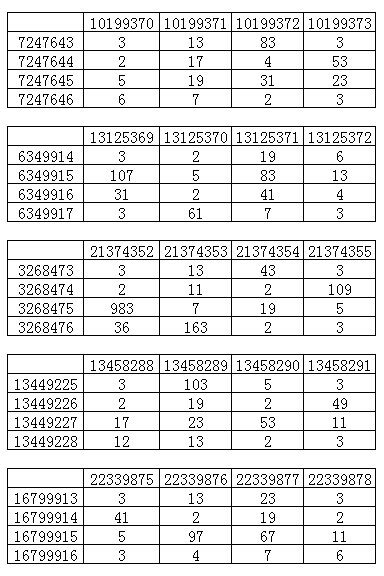并且发现已经有人捷足先登了.
Fans继续努力，程序经过通宵运行，找出n=5的解:
x=7759032126,y=247376414072

y y+1 y+2 y+3 y+4
x 2 3 2 41 2
x+1 137 37 103 7 11
x+2 2 19 2 29 2
x+3 23 3 109 31 3
x+4 2 47 2 5 2

x=14150396180,y=232889465522

y y+1 y+2 y+3 y+4
x 2 37 2 5 2
x+1 79 3 67 97 3
x+2 2 13 2 17 2
x+3 7 11 31 83 43
x+4 2 3 2 29 3

x=37945747188,y=131487690152

y y+1 y+2 y+3 y+4
x 2 3 2 73 2
x+1 7 41 277 151 109
x+2 2 89 2 5 2
x+3 31 3 83 19 3
x+4 2 17 2 409 2

Github